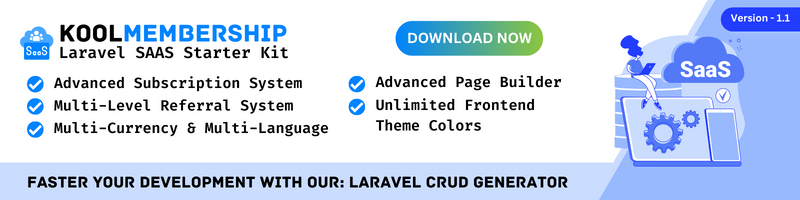In this post, I will show you how to get a table name using the model and the table columns in Laravel 8. If you need to generate the table name using the model and display the table columns, this post is for you.

Currently, I'm doing a task that will get the assigned table in the model and display the table columns.

So here is the code on how to do it. In your controller method add this code.

``````/**
* Display a listing of the resource.
* @return Renderable
*/
public function index()
{
\$model = '\App\Models\User';

if(method_exists(\$model, 'getTable')) {
// Get table name using model
\$tableName = (new \$model)->getTable();
// Get table columns
\$tableColumns = Schema::getColumnListing(\$tableName);

print_r(\$tableColumns);
}
}``````

Result:

``````Array
(
 => id
 => name
 => first_name
 => last_name
 => email
 => email_verified_at
 => created_at
 => updated_at
)
``````

Now you have an idea of how to extract the model table name and get all columns into it. I hope it helps :)